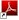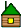## Европейская школа по Физике высоких энергий (ESHEP-2019) 2019 EUROPEAN SCHOOL OF HIGH-ENERGY PHYSICS

Roger Barlow
Practical Statistics

Lecture 1: The BasicsProbability
• What is it?
• Frequentist Probability
• Conditional Probability and Bayes’ Theorem
• Bayesian Probability

Probability distributions and their properties

• Expectation Values
• Binomial, Poisson and Gaussian

Hypothesis testing

Lecture 2: Estimation and ErrorsEstimation
• Bias
• Efficiency
• Maximum Likelihood Estimation
• Least squares
• Straight line fits
• Fitting Histograms

Errors
• Errors from Likelihood
• Asymmetric Errors
• Systematic Errors

Lecture 3: Setting Limits and making discoveriesGoodness of Fit
• p−values
• The χ2 distribution
• Wilks’ Theorerm
• Toy Monte Carlos and Likelihood for Goodness of Fit
Upper Limits
• Frequentist Confidence
• Confidence Belts
• Coverage
• Bayesian Intervals
• Feldman-Cousins
• CLS

Making a Discovery

• Sigma language
• The Look Elsewhere Effect
• Blind Analysis

Conclusions

Alexander Bednyakov
Field Theory and the E-W Standard Model

• What is the Standard Model?
•  Introducing Quantum Fields
• Interactions and Perturbation Theory
•  Renormalizable or Non-Renormalizable?

• An Ode to Symmetry
• Global Symmetries (and Conserved Quantities)
• Local Symmetries (and Gauge interactions)
• From Fermi Model to EW theory

• Finalizing the EW SM (a bit of Higgsing)
• Features” of the SM
• Experimental tests of the EW
• SM Issues and Prospects of the EW SM

Jamie Boyd
LHC Run-2 and Future ProspectsBrief discussion on LHC
Highlights from LHC results in Run-2, and future prospects

• Mostly ATLAS/CMS but also LHCb
• Will not show heavy ion results, so ALICE not covered
• Try to show the diversity of LHC physics and the versatility of the LHC and detectors
• Try to mention various experimental innovations where relevant

Jo van den Brand
Special Lecture on Gravitational WavesJohn Ellis
Higgs Physics

Concha Gonzalez-Garcia

Neutrino Physics

• Historic Introduction
• Neutrinos in the SM
• Neutrino Properties: Helicity versus Chirality, Majorana versus Dirac
• Neutrino Mass Terms Beyond the SM: Dirac, Majorana, the See-Saw Mechanisms, Lepton Mixing
• Probes of Neutrino Mass Scale
• Neutrino Oscillations in Vacuum
• Experimental Probes
• Atmospheric Neutrinos
• Accelerator Neutrinos at Long Baselines
• Reactor Neutrinos
• Solar Neutrinos
• Flavour Transitions in Matter: MSW

Lecture III• Fitting all Together: The New Minimal Standard Model
• Some Implications

Valery Rubakov
Cosmology and Dark Matter

• Expanding Universe
• Dark matter: evidence
• WIMPs

• WIMP search
• Dark matter axions
• Theory
• Cosmology
• Search
• Fuzzy dark matter
• Warm dark matter
• Sterile neutrino
• Gravitino
• Dark matter summary

• Baryon asymmetry of the Universe
• Generalities.
• Electroweak baryon number non-conservation
• What can make electroweak mechanism work?
• Leptogenesis and neutrino masses
• Before the hot epoch

Veronica Sanz
Physics Beyond the Standard Model

1. Evidence (DM, Neutrinos, Baryogenesis & Inflation )
2. Rationale (Example of Naturalness)
3. Models for the Higgs and beyond (Supersymmetry & Composite Higgs)

Mikhail Vysotsky
Flavour Physics and CP Violation

Lecture I + Lecture II + Lecture IIIGiulia Zanderigh
QCD

Perturbative QCD as a tool for precision QCD at colliders

• Introduction to QCD (or refresh your knowledge of QCD)
• Basic concepts which appear over and over in different contexts
• Understand the terminology
• Recent developments in the field

In the following we will concentrate on the perturbative regime of QCD.
In particular, we’ll discuss generic properties of QCD amplitudes

• Soft-collinear divergences (and how they are dealt with)
• Kinoshita-Lee-Nauenberg theorem
• The concept of infrared finiteness
• Sterman Weinberg jets

Lecture IIILHC kinematics

Lecture IVjets
Monte Carlos

Korinna Zapp

Heavy-Ion Physics

go through dierent stages of heavy ion collisions
focus on soft particle production

• hard processes
• quarkonia
• electroweak bosons
• jets
• small collision systems
• other fun stuff with heavy ions